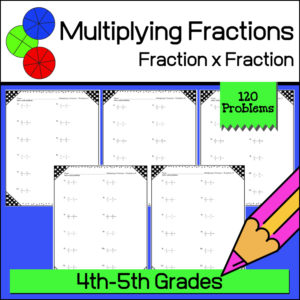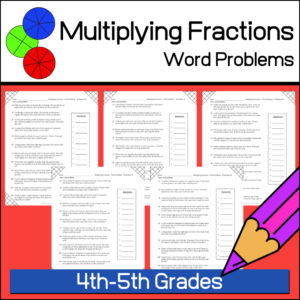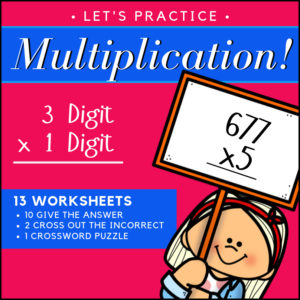Showing all 15 results

•\$3.00

This resource is designed to give students the practice the need to become proficient at multiplying decimals. There are three levels, 15 worksheets (16 problems each) and answer keys. That’s 240 problems – EACH WORKSHEET includes a different growth mindset quote!
(See description below for more details)

•\$3.00

This resource is designed to give students the practice the need to become proficient at multiplying decimal numbers. There are three levels, 15 worksheets (20 problems each) and answer keys. That’s 300 problems! Use as practice sheets, homework, or for quizzes and tests.

Also included: Visual poster to help students with ‘where to place’ the decimal point in their answers.

3 Levels:

• Level 1 (5 worksheets)
Tenths multiplied by tenths
• Level 2 (5 worksheets)
Tenths multiplied by hundreds and/or by thousandths
Hundredths multiplied by hundredths and/or by thousandths
• Level 3 (5 worksheets)
Mixed from tenths/hundredths/thousandths multiplied by tenths/hundredths/thousandths
•\$3.00

Give your students the practice and reinforcement they need as they are learning to multiply fractions with fractions! This resource includes 120 problems (10 worksheets with 12 problems each) and answer keys.

•\$3.00

Help students develop their math word problem skills and practice solving multiplying fractions all at the same time! This resources provides ten (10) ready to use, print and go worksheets each with 12 word problems for a total of 120 problems (answer keys provided).

•\$2.50

Give your students the practice and reinforcement they need as they are learning to multiply fractions by whole numbers! This resource includes 100 problems (5 worksheets with 20 problems each) and answer keys.

•\$3.00

Help your students understand and remember the basis of multiplying fractions using a visual model method! This product, Multiplying Fractions with Visual Models #2, gives you 110 problems in which students will multiply a fraction with a another fraction. For each problem students will be asked to color in given visual fraction models to find the answer before giving the answer.

Product includes 10 worksheets (11 questions each) = 110 questions + answer keys.

•\$2.25
•\$3.00

Help your students understand and remember the basis of multiplying fractions using a visual model method! This product gives you 120 problems in which students will multiply a fraction with a whole number. For each problem students will be asked to color in given visual fraction models to find the answer. Then they are to write the answers using mixed numbers.

Product includes 10 worksheets (12 questions each) = 120 questions + answer keys.

•\$4.99

Give your students a fun way to practice their multiplication facts! Your students will love this fast paced math game with included fun twists such as: the ‘Green Horn‘, ‘Stampede!’, ‘Playing in the Mud‘, ‘Stomped‘ and ‘Growing Herd‘. Your students are going to want to play this game again and again!

Includes:

• – Game instructions
• – Game Cards (all facts 0-12 PLUS fun ‘twist’ game cards)
• – (Optional) Card backs
•\$2.25

These 300 problems will give your students the practice they need to reinforce their multiplication skills (single digit number x double digit)! This resource includes 10 worksheets with 30 problems each. Each can be used by students for practice or for assessment (quiz and test). Answer keys provided.

Skill: Students will multiply a two digit whole number by a one-digit whole number.

•\$4.99

OVER 1,800 problems, your students will have the practice they need to understand and learn their multiplication facts 0 to 10!

The resource begins with repeated addition (to reinforce the concept of addition in relation to multiplication) and ends with students completing all multiplication facts.

•\$2.25

These 300 problems will give your students the practice they need to reinforce their multiplication skills (double digit x double digit)! This resource includes 15 worksheets with 20 problems each. Each can be used by students for practice or for assessment (quiz and test). Answer keys provided.

Skill: Students will multiply a double digit whole number by a double-digit whole number.

•\$2.00

Give your students the practice they need to reinforce and demonstrate fluency in multiplication with factors 0-12. This resource includes 200 problems – 10 worksheets with 20 problems each.

You can use as ‘normal’ worksheets or use in timed drills! Answer keys provided.

•\$2.50

This resource includes 10 worksheets (each with 12 problems). Students will be asked to identify factors and multiples of given numbers.

•\$3.00

These 500 problems will give your students the practice they need to reinforce their multiplication skills (triple digit x single – double – triple digit)! Can be used by students for practice or for assessment (quiz and test). Answer keys provided.

Skill: Students will multiply a triple digit whole number by a single, double or triple digit whole number.

Includes:

• – 5 Worksheets (triple x single digit) 125 problems total
• – 5 Worksheets (triple x double digit) 100 problems total
• – 5 Worksheets (triple x triple digit) 75 problems total
• – 5 Worksheets (mixed – triple x single, double and triple digit) 75 problems total